JalFaizy Shaikh — Published On January 28, 2019 and Last Modified On June 20th, 2022

## Introduction

Humans do not reboot their understanding of language each time we hear a sentence. Given an article, we grasp the context based on our previous understanding of those words. One of the defining characteristics we possess is our memory (or retention power).

Can an algorithm replicate this? The first technique that comes to mind is a neural network (NN). But the traditional NNs unfortunately cannot do this. Take an example of wanting to predict what comes next in a video. A traditional neural network will struggle to generate accurate results.

That’s where the concept of recurrent neural networks (RNNs) comes into play. RNNs have become extremely popular in the deep learning space which makes learning them even more imperative. A few real-world applications of RNN include:

• Speech recognition
• Machine translation
• Music composition
• Handwriting recognition
• Grammar learning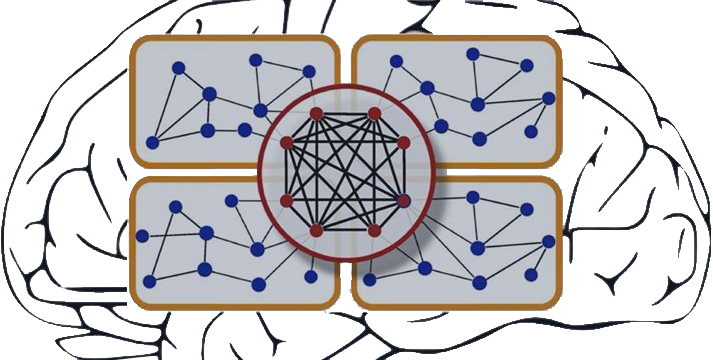In this article, we’ll first quickly go through the core components of a typical RNN model. Then we’ll set up the problem statement which we will finally solve by implementing an RNN model from scratch in Python.

We can always leverage high-level Python libraries to code a RNN. So why code it from scratch? I firmly believe the best way to learn and truly ingrain a concept is to learn it from the ground up. And that’s what I’ll showcase in this tutorial.

This article assumes a basic understanding of recurrent neural networks. In case you need a quick refresher or are looking to learn the basics of RNN, I recommend going through the below articles first:

• Flashback: A Recap of Recurrent Neural Network Concepts
• Sequence Prediction using RNN
• Building an RNN Model using Python

## Flashback: A Recap of Recurrent Neural Network Concepts

Let’s quickly recap the core concepts behind recurrent neural networks.

We’ll do this using an example of sequence data, say the stocks of a particular firm. A simple machine learning model, or an Artificial Neural Network, may learn to predict the stock price based on a number of features, such as the volume of the stock, the opening value, etc. Apart from these, the price also depends on how the stock fared in the previous fays and weeks. For a trader, this historical data is actually a major deciding factor for making predictions.

In conventional feed-forward neural networks, all test cases are considered to be independent. Can you see how that’s a bad fit when predicting stock prices? The NN model would not consider the previous stock price values – not a great idea!

There is another concept we can lean on when faced with time sensitive data – Recurrent Neural Networks (RNN)!

A typical RNN looks like this: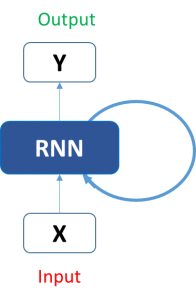This may seem intimidating at first. But once we unfold it, things start looking a lot simpler: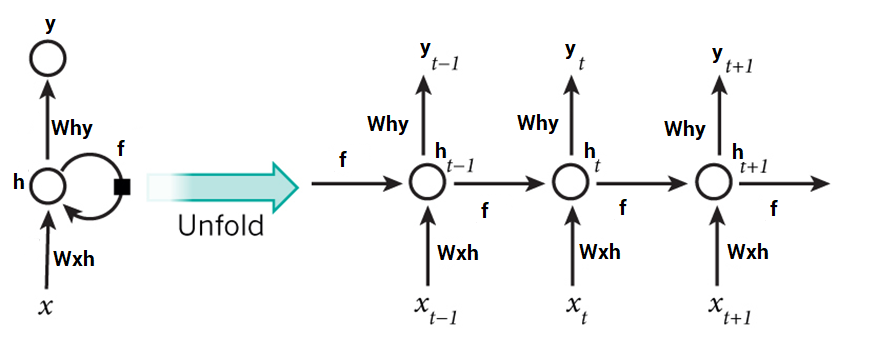It is now easier for us to visualize how these networks are considering the trend of stock prices. This helps us in predicting the prices for the day. Here, every prediction at time t (h_t) is dependent on all previous predictions and the information learned from them. Fairly straightforward, right?

RNNs can solve our purpose of sequence handling to a great extent but not entirely.

Text is another good example of sequence data. Being able to predict what word or phrase comes after a given text could be a very useful asset. We want our models to write Shakespearean sonnets!

Now, RNNs are great when it comes to context that is short or small in nature. But in order to be able to build a story and remember it, our models should be able to understand the context behind the sequences, just like a human brain.

## Sequence Prediction using RNN

In this article, we will work on a sequence prediction problem using RNN. One of the simplest tasks for this is sine wave prediction. The sequence contains a visible trend and is easy to solve using heuristics. This is what a sine wave looks like: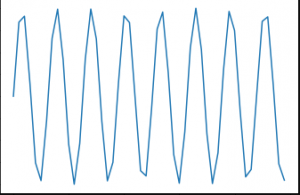We will first devise a recurrent neural network from scratch to solve this problem. Our RNN model should also be able to generalize well so we can apply it on other sequence problems.

We will formulate our problem like this – given a sequence of 50 numbers belonging to a sine wave, predict the 51st number in the series. Time to fire up your Jupyter notebook (or your IDE of choice)!

## Coding RNN using Python

### Step 0: Data Preparation

Ah, the inevitable first step in any data science project – preparing the data before we do anything else.

What does our network model expect the data to be like? It would accept a single sequence of length 50 as input. So the shape of the input data will be:

`(number_of_records x length_of_sequence x types_of_sequences)`

Here, types_of_sequences is 1, because we have only one type of sequence – the sine wave.

On the other hand, the output would have only one value for each record. This will of course be the 51st value in the input sequence. So it’s shape would be:

`(number_of_records x types_of_sequences) #where types_of_sequences is 1`

Let’s dive into the code. First, import the necessary libraries:

```%pylab inline

import math```

To create a sine wave like data, we will use the sine function from Python’s math library:

`sin_wave = np.array([math.sin(x) for x in np.arange(200)])`

Visualizing the sine wave we’ve just generated:

`plt.plot(sin_wave[:50])`We will create the data now in the below code block and print the shape of the data:

Python Code:

Note that we looped for (num_records – 50) because we want to set aside 50 records as our validation data. We can create this validation data now:
```X_val = []
Y_val = []

for i in range(num_records - 50, num_records):
X_val.append(sin_wave[i:i+seq_len])
Y_val.append(sin_wave[i+seq_len])

X_val = np.array(X_val)
X_val = np.expand_dims(X_val, axis=2)

Y_val = np.array(Y_val)
Y_val = np.expand_dims(Y_val, axis=1)```

### Step 1: Create the Architecture for our RNN model

Our next task is defining all the necessary variables and functions we’ll use in the RNN model. Our model will take in the input sequence, process it through a hidden layer of 100 units, and produce a single valued output:

```learning_rate = 0.0001
nepoch = 25
T = 50                   # length of sequence
hidden_dim = 100
output_dim = 1

bptt_truncate = 5
min_clip_value = -10
max_clip_value = 10```

We will then define the weights of the network:

```U = np.random.uniform(0, 1, (hidden_dim, T))
W = np.random.uniform(0, 1, (hidden_dim, hidden_dim))
V = np.random.uniform(0, 1, (output_dim, hidden_dim))```

Here,

• U is the weight matrix for weights between input and hidden layers
• V is the weight matrix for weights between hidden and output layers
• W is the weight matrix for shared weights in the RNN layer (hidden layer)

Finally, we will define the activation function, sigmoid, to be used in the hidden layer:

```def sigmoid(x):
return 1 / (1 + np.exp(-x))```

### Step 2: Train the Model

Now that we have defined our model, we can finally move on with training it on our sequence data. We can subdivide the training process into smaller steps, namely:

Step 2.1 : Check the loss on training data
Step 2.1.1 : Forward Pass
Step 2.1.2 : Calculate Error
Step 2.2 : Check the loss on validation data
Step 2.2.1 : Forward Pass
Step 2.2.2 : Calculate Error
Step 2.3 : Start actual training
Step 2.3.1 : Forward Pass
Step 2.3.2 : Backpropagate Error
Step 2.3.3 : Update weights

We need to repeat these steps until convergence. If the model starts to overfit, stop! Or simply pre-define the number of epochs.

#### Step 2.1: Check the loss on training data

We will do a forward pass through our RNN model and calculate the squared error for the predictions for all records in order to get the loss value.

```for epoch in range(nepoch):
# check loss on train
loss = 0.0

# do a forward pass to get prediction
for i in range(Y.shape):
x, y = X[i], Y[i]                    # get input, output values of each record
prev_s = np.zeros((hidden_dim, 1))   # here, prev-s is the value of the previous activation of hidden layer; which is initialized as all zeroes
for t in range(T):
new_input = np.zeros(x.shape)    # we then do a forward pass for every timestep in the sequence
new_input[t] = x[t]              # for this, we define a single input for that timestep
mulu = np.dot(U, new_input)
mulw = np.dot(W, prev_s)
mulv = np.dot(V, s)
prev_s = s

# calculate error
loss_per_record = (y - mulv)**2 / 2
loss += loss_per_record
loss = loss / float(y.shape)```

#### Step 2.2: Check the loss on validation data

We will do the same thing for calculating the loss on validation data (in the same loop):

```    # check loss on val
val_loss = 0.0
for i in range(Y_val.shape):
x, y = X_val[i], Y_val[i]
prev_s = np.zeros((hidden_dim, 1))
for t in range(T):
new_input = np.zeros(x.shape)
new_input[t] = x[t]
mulu = np.dot(U, new_input)
mulw = np.dot(W, prev_s)
mulv = np.dot(V, s)
prev_s = s

loss_per_record = (y - mulv)**2 / 2
val_loss += loss_per_record
val_loss = val_loss / float(y.shape)

print('Epoch: ', epoch + 1, ', Loss: ', loss, ', Val Loss: ', val_loss)```

You should get the below output:

```Epoch:  1 , Loss:  [[101185.61756671]] , Val Loss:  [[50591.0340148]]
...
...```

#### Step 2.3: Start actual training

We will now start with the actual training of the network. In this, we will first do a forward pass to calculate the errors and a backward pass to calculate the gradients and update them. Let me show you these step-by-step so you can visualize how it works in your mind.

#### Step 2.3.1: Forward Pass

In the forward pass:

• We first multiply the input with the weights between input and hidden layers
• Add this with the multiplication of weights in the RNN layer. This is because we want to capture the knowledge of the previous timestep
• Pass it through a sigmoid activation function
• Multiply this with the weights between hidden and output layers
• At the output layer, we have a linear activation of the values so we do not explicitly pass the value through an activation layer
• Save the state at the current layer and also the state at the previous timestep in a dictionary

Here is the code for doing a forward pass (note that it is in continuation of the above loop):

```    # train model
for i in range(Y.shape):
x, y = X[i], Y[i]

layers = []
prev_s = np.zeros((hidden_dim, 1))
dU = np.zeros(U.shape)
dV = np.zeros(V.shape)
dW = np.zeros(W.shape)

dU_t = np.zeros(U.shape)
dV_t = np.zeros(V.shape)
dW_t = np.zeros(W.shape)

dU_i = np.zeros(U.shape)
dW_i = np.zeros(W.shape)

# forward pass
for t in range(T):
new_input = np.zeros(x.shape)
new_input[t] = x[t]
mulu = np.dot(U, new_input)
mulw = np.dot(W, prev_s)
mulv = np.dot(V, s)
layers.append({'s':s, 'prev_s':prev_s})
prev_s = s
```

#### Step 2.3.2 : Backpropagate Error

After the forward propagation step, we calculate the gradients at each layer, and backpropagate the errors. We will use truncated back propagation through time (TBPTT), instead of vanilla backprop. It may sound complex but its actually pretty straight forward.

The core difference in BPTT versus backprop is that the backpropagation step is done for all the time steps in the RNN layer. So if our sequence length is 50, we will backpropagate for all the timesteps previous to the current timestep.

If you have guessed correctly, BPTT seems very computationally expensive. So instead of backpropagating through all previous timestep , we backpropagate till x timesteps to save computational power. Consider this ideologically similar to stochastic gradient descent, where we include a batch of data points instead of all the data points.

Here is the code for backpropagating the errors:

```        # derivative of pred
dmulv = (mulv - y)

# backward pass
for t in range(T):
dV_t = np.dot(dmulv, np.transpose(layers[t]['s']))
dsv = np.dot(np.transpose(V), dmulv)

ds = dsv

dprev_s = np.dot(np.transpose(W), dmulw)

for i in range(t-1, max(-1, t-bptt_truncate-1), -1):
ds = dsv + dprev_s

dW_i = np.dot(W, layers[t]['prev_s'])
dprev_s = np.dot(np.transpose(W), dmulw)

new_input = np.zeros(x.shape)
new_input[t] = x[t]
dU_i = np.dot(U, new_input)
dx = np.dot(np.transpose(U), dmulu)

dU_t += dU_i
dW_t += dW_i

dV += dV_t
dU += dU_t
dW += dW_t```

#### Step 2.3.3 : Update weights

Lastly, we update the weights with the gradients of weights calculated. One thing we have to keep in mind that the gradients tend to explode if you don’t keep them in check.This is a fundamental issue in training neural networks, called the exploding gradient problem. So we have to clamp them in a range so that they dont explode. We can do it like this

```            if dU.max() > max_clip_value:
dU[dU > max_clip_value] = max_clip_value
if dV.max() > max_clip_value:
dV[dV > max_clip_value] = max_clip_value
if dW.max() > max_clip_value:
dW[dW > max_clip_value] = max_clip_value

if dU.min() < min_clip_value:
dU[dU < min_clip_value] = min_clip_value
if dV.min() < min_clip_value:
dV[dV < min_clip_value] = min_clip_value
if dW.min() < min_clip_value:
dW[dW < min_clip_value] = min_clip_value

# update
U -= learning_rate * dU
V -= learning_rate * dV
W -= learning_rate * dW```

On training the above model, we get this output:

```Epoch:  1 , Loss:  [[101185.61756671]] , Val Loss:  [[50591.0340148]]
Epoch:  2 , Loss:  [[61205.46869629]] , Val Loss:  [[30601.34535365]]
Epoch:  3 , Loss:  [[31225.3198258]] , Val Loss:  [[15611.65669247]]
Epoch:  4 , Loss:  [[11245.17049551]] , Val Loss:  [[5621.96780111]]
Epoch:  5 , Loss:  [[1264.5157739]] , Val Loss:  [[632.02563908]]
Epoch:  6 , Loss:  [[20.15654115]] , Val Loss:  [[10.05477285]]
Epoch:  7 , Loss:  [[17.13622839]] , Val Loss:  [[8.55190426]]
Epoch:  8 , Loss:  [[17.38870495]] , Val Loss:  [[8.68196484]]
Epoch:  9 , Loss:  [[17.181681]] , Val Loss:  [[8.57837827]]
Epoch:  10 , Loss:  [[17.31275313]] , Val Loss:  [[8.64199652]]
Epoch:  11 , Loss:  [[17.12960034]] , Val Loss:  [[8.54768294]]
Epoch:  12 , Loss:  [[17.09020065]] , Val Loss:  [[8.52993502]]
Epoch:  13 , Loss:  [[17.17370113]] , Val Loss:  [[8.57517454]]
Epoch:  14 , Loss:  [[17.04906914]] , Val Loss:  [[8.50658127]]
Epoch:  15 , Loss:  [[16.96420184]] , Val Loss:  [[8.46794248]]
Epoch:  16 , Loss:  [[17.017519]] , Val Loss:  [[8.49241316]]
Epoch:  17 , Loss:  [[16.94199493]] , Val Loss:  [[8.45748739]]
Epoch:  18 , Loss:  [[16.99796892]] , Val Loss:  [[8.48242177]]
Epoch:  19 , Loss:  [[17.24817035]] , Val Loss:  [[8.6126231]]
Epoch:  20 , Loss:  [[17.00844599]] , Val Loss:  [[8.48682234]]
Epoch:  21 , Loss:  [[17.03943262]] , Val Loss:  [[8.50437328]]
Epoch:  22 , Loss:  [[17.01417255]] , Val Loss:  [[8.49409597]]
Epoch:  23 , Loss:  [[17.20918888]] , Val Loss:  [[8.5854792]]
Epoch:  24 , Loss:  [[16.92068017]] , Val Loss:  [[8.44794633]]
Epoch:  25 , Loss:  [[16.76856238]] , Val Loss:  [[8.37295808]]```

Looking good! Time to get the predictions and plot them to get a visual sense of what we’ve designed.

### Step 3: Get predictions

We will do a forward pass through the trained weights to get our predictions:

```preds = []
for i in range(Y.shape):
x, y = X[i], Y[i]
prev_s = np.zeros((hidden_dim, 1))
# Forward pass
for t in range(T):
mulu = np.dot(U, x)
mulw = np.dot(W, prev_s)
mulv = np.dot(V, s)
prev_s = s

preds.append(mulv)

preds = np.array(preds)```

Plotting these predictions alongside the actual values:

```plt.plot(preds[:, 0, 0], 'g')
plt.plot(Y[:, 0], 'r')
plt.show()```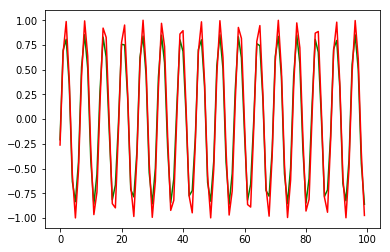This was on the training data. How do we know if our model didn’t overfit? This is where the validation set, which we created earlier, comes into play:
```preds = []
for i in range(Y_val.shape):
x, y = X_val[i], Y_val[i]
prev_s = np.zeros((hidden_dim, 1))
# For each time step...
for t in range(T):
mulu = np.dot(U, x)
mulw = np.dot(W, prev_s)
mulv = np.dot(V, s)
prev_s = s

preds.append(mulv)

preds = np.array(preds)

plt.plot(preds[:, 0, 0], 'g')
plt.plot(Y_val[:, 0], 'r')
plt.show()```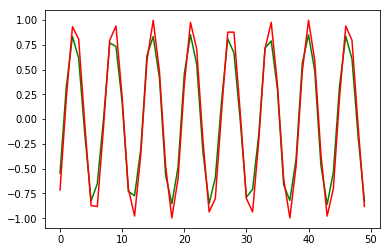Not bad. The predictions are looking impressive. The RMSE score on the validation data is respectable as well:
```from sklearn.metrics import mean_squared_error

math.sqrt(mean_squared_error(Y_val[:, 0] * max_val, preds[:, 0, 0] * max_val))
```
`0.127191931509431`

## End Notes

I cannot stress enough how useful RNNs are when working with sequence data. I implore you all to take this learning and apply it on a dataset. Take a NLP problem and see if you can find a solution for it. You can always reach out to me in the comments section below if you have any questions.

In this article, we learned how to create a recurrent neural network model from scratch by using just the numpy library. You can of course use a high-level library like Keras or Caffe but it is essential to know the concept you’re implementing.###### JalFaizy Shaikh

Faizan is a Data Science enthusiast and a Deep learning rookie. A recent Comp. Sc. undergrad, he aims to utilize his skills to push the boundaries of AI research.

## 12 thoughts on "Build a Recurrent Neural Network from Scratch in Python – An Essential Read for Data Scientists"###### Guy Aubin says:January 30, 2019 at 3:03 am
Great article! How would the code need to be modified if more than one time series are used to make a prediction? For example: - predict next day temperature using the last 50-day temperature and last 50-day humidity level; or, - predict next day temperature and next day humidity level using the last 50-day temperature and last 50-day humidity level Thank you, Guy Aubin Reply###### Pulkit Sharma says:January 31, 2019 at 12:34 pm
Hi, As the data for both the tasks, i.e. predicting temperature and predicting humidity, will be different, I would suggest to build two different models, one for temperature and one for humidity. Reply###### Guy Aubin says:January 31, 2019 at 5:35 pm
@pulkit_sharma. Thank you Pulkit. But still, future temperature can be correlated to both past temperature and past humidity level, so incorporating both variables as inputs will improve the output; how would you incorporate two variables in the model. Also, is it possible to have a single model make the prediction for both the temperature and humidity since these variables are correlated? Reply###### HALA says:May 25, 2019 at 11:08 am
Hi How can I work it With audio Data Reply###### Aishwarya Singh says:May 27, 2019 at 11:08 am###### Zhenxing says:December 02, 2021 at 7:08 pm
``` # derivative of pred dmulv = (mulv - y) # backward pass for t in range(T): dV_t = np.dot(dmulv, np.transpose(layers[t]['s'])) dsv = np.dot(np.transpose(V), dmulv) ``` quick question here: can we back propagate the derivative of prediction loss back to every t in the sequence in the `many-to-one` setting? Reply###### Thai Tran says:December 06, 2021 at 5:54 am
at the end: what is max_val? Reply###### Meharunnisa says:July 18, 2022 at 3:00 pm
Can we use the same code for DNA Sequence? Reply###### Youngseok Choi says:September 14, 2022 at 9:34 am
Above End Notes, in the equations what is the max_val? Reply###### Laine says:October 15, 2022 at 10:39 pm
Thanks for the tutorial. How would we include static data as well as sequential data? Reply###### John says:December 30, 2022 at 5:40 pm
Hello, I'm very interested in the neural network code. However, the code on replit does not load. How else can you see this code? Reply###### Charlie says:February 26, 2023 at 2:55 pm
Is there some reason why loss_per_record = (y - mulv)**2 / 2, not that loss_per_record = (y - mulv)**2 ? Reply